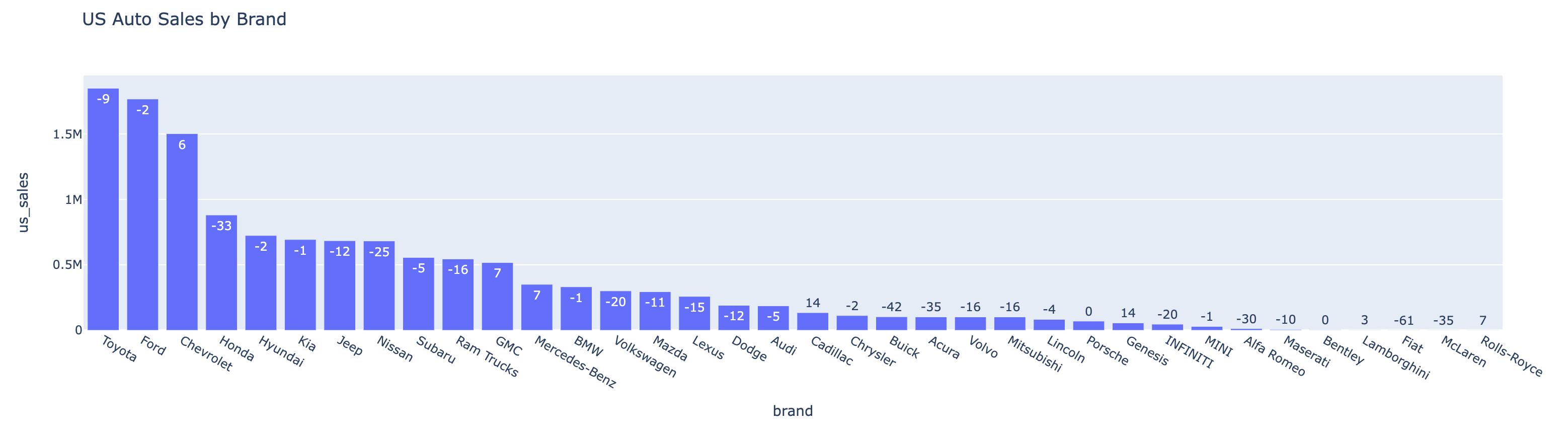# Plot

## API

``````DataFrame.ai.plot(desc: Optional[str] = None, cache: bool = True) -> str
``````
This method is used to plot a Spark DataFrame, the specifics of which are determined by the `desc` parameter. If `desc` is not provided, the method will try to plot the DataFrame based on its schema.

• param desc: An optional natural language string that outlines the specific transformation to be applied on the DataFrame.
• param cache: If `True`, fetches cached data, if available. If `False`, retrieves fresh data and updates cache.
• return: Returns the generated code as a string. If the generated code is not valid Python code, an empty string is returned.

## Example

Given a DataFrame `auto_df` from Data Ingestion, you can plot it with the following code:

``````auto_df.ai.plot()
``````To plot with an instruction:

``````auto_df.ai.plot("pie chart for US sales market shares, show the top 5 brands and the sum of others")
``````